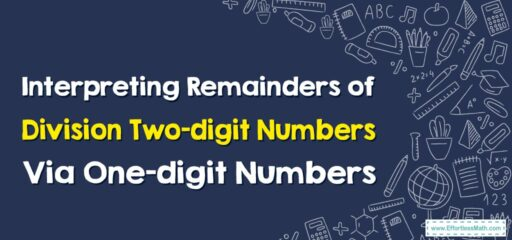# How to Interpret Remainders of Division Two-digit Numbers By One-digit Numbers

When you divide two-digit numbers by one-digit numbers, you may often end up with a remainder. This means that the two-digit number cannot be divided evenly by the one-digit number.## A Step-by-step Guide to Interpreting Remainders of Division Two-digit Numbers By One-digit Numbers

Here’s a step-by-step guide using an example problem:

### Step 1: Perform the Division

The first step is to perform the division. Divide 67 by 8.

### Step 2: Calculate the Quotient and Remainder

When you divide 67 by 8, you get a quotient of 8 and a remainder of 3. This means that you can distribute 67 items into 8 equal groups with 3 items left over.

### Step 3: Interpret the Quotient

The quotient is the number of whole times that 8 can fit into 67. In other words, you can make 8 full groups of 8 from the number 67.

### Step 4: Interpret the Remainder

The remainder is the amount left over after all the full groups of 8 have been taken out of 67. So, after making 8 groups of 8, you would have 3 left over.

### Step 5: Contextualize the Remainder

Depending on the context of the problem, the remainder can have different interpretations. For instance, if you were trying to equally distribute 67 candies among 8 children, the remainder of 3 would represent the candies that could not be evenly distributed. Each child would get 8 candies, and 3 candies would be left over.

### Step 6: Express the Result in Different Ways

Depending on your needs, you can express the result of the division in different ways. For example:

• As a mixed number: 8 remainder 3 can be written as $$8\frac{3}{8}$$.
• As a decimal: Perform further division to turn the remainder into a decimal. Divide the remainder of 3 by the divisor 8 to get 0.375. So, 67 divided by 8 is 8.375.
• As a fraction: The remainder can be written as a fraction over the divisor. So, 8 remainders 3 would be $$8\frac{3}{8}$$.

Remember, the interpretation of the remainder should make sense in the context of the problem you’re solving.

### What people say about "How to Interpret Remainders of Division Two-digit Numbers By One-digit Numbers - Effortless Math: We Help Students Learn to LOVE Mathematics"?

No one replied yet.

X
30% OFF

Limited time only!

Save Over 30%

SAVE $5 It was$16.99 now it is \$11.99